•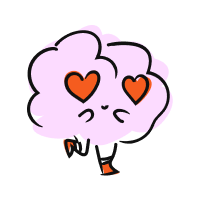• Merch
• Donate
• SAT Prep

IntroductionIntroduction# Decimals

INTRO
A decimal is a number that has a whole part and decimal part that are separated by a decimal point.
Each digit in a decimal number has a place value, which tells us how big or small a digit in a decimal number is based on its distance and direction from the decimal point.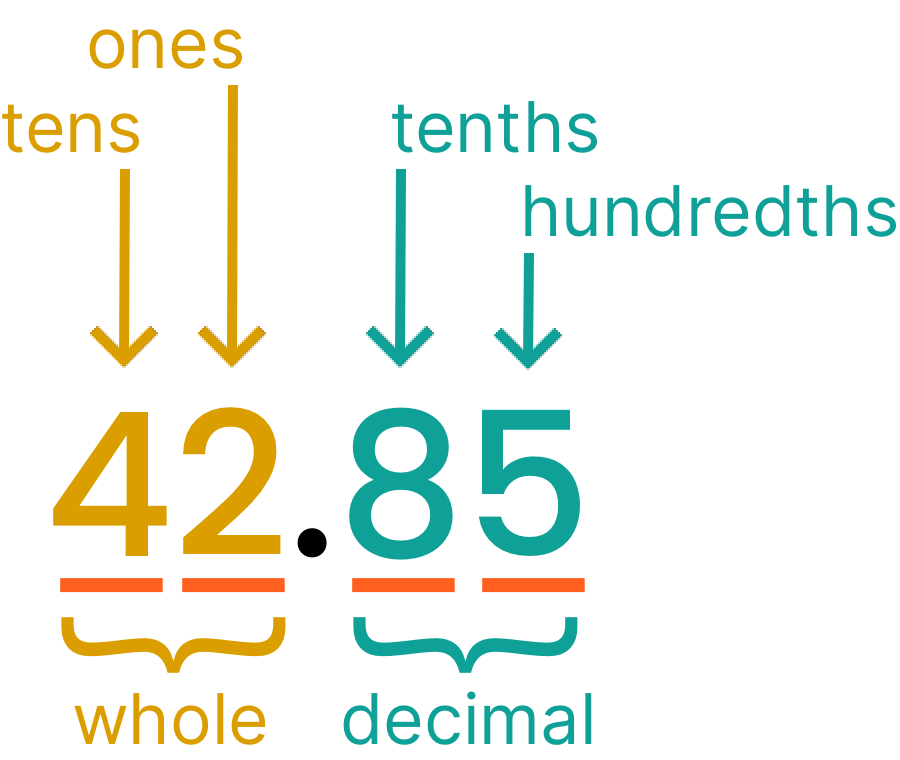As we move from the place value gets divided by .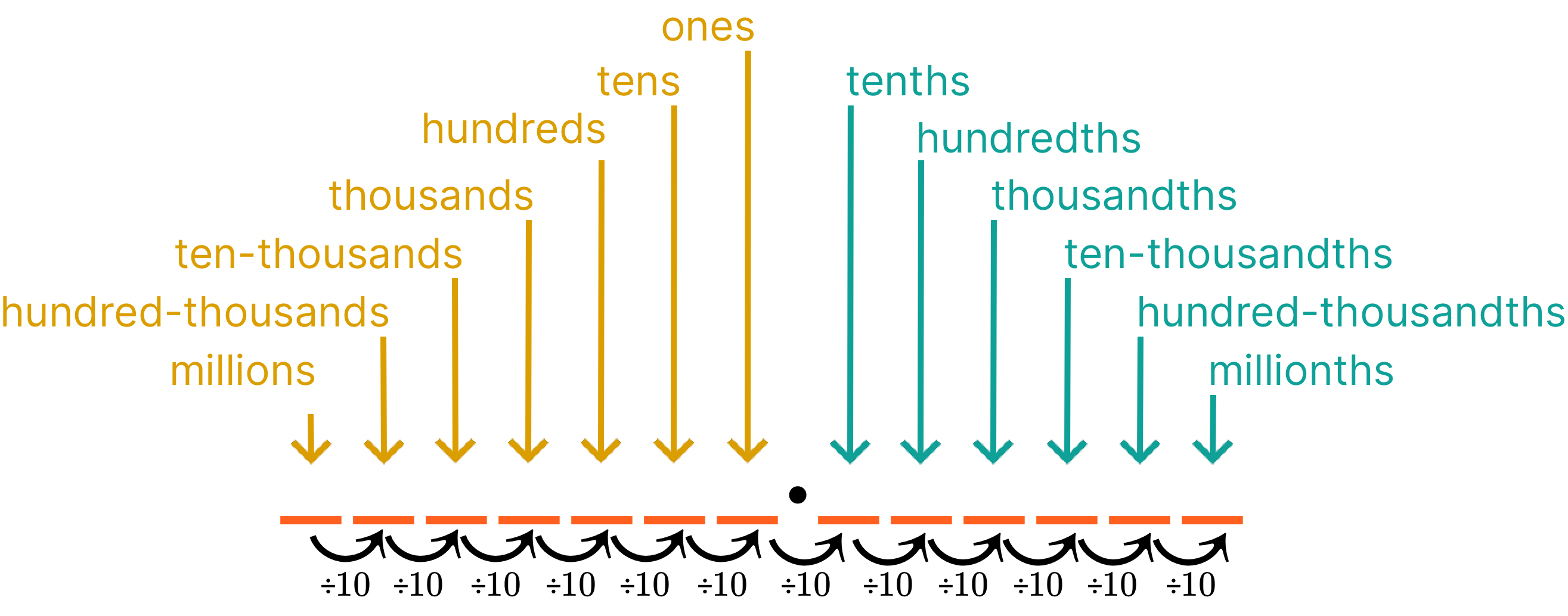In order to better understand decimals and place values, let’s imagine that we have a cash register that only has bills and coins that are powers of .
Let’s say we needed to give a customer of change using the fewest bills and coins possible. How many of each bill and coin would we need to give them?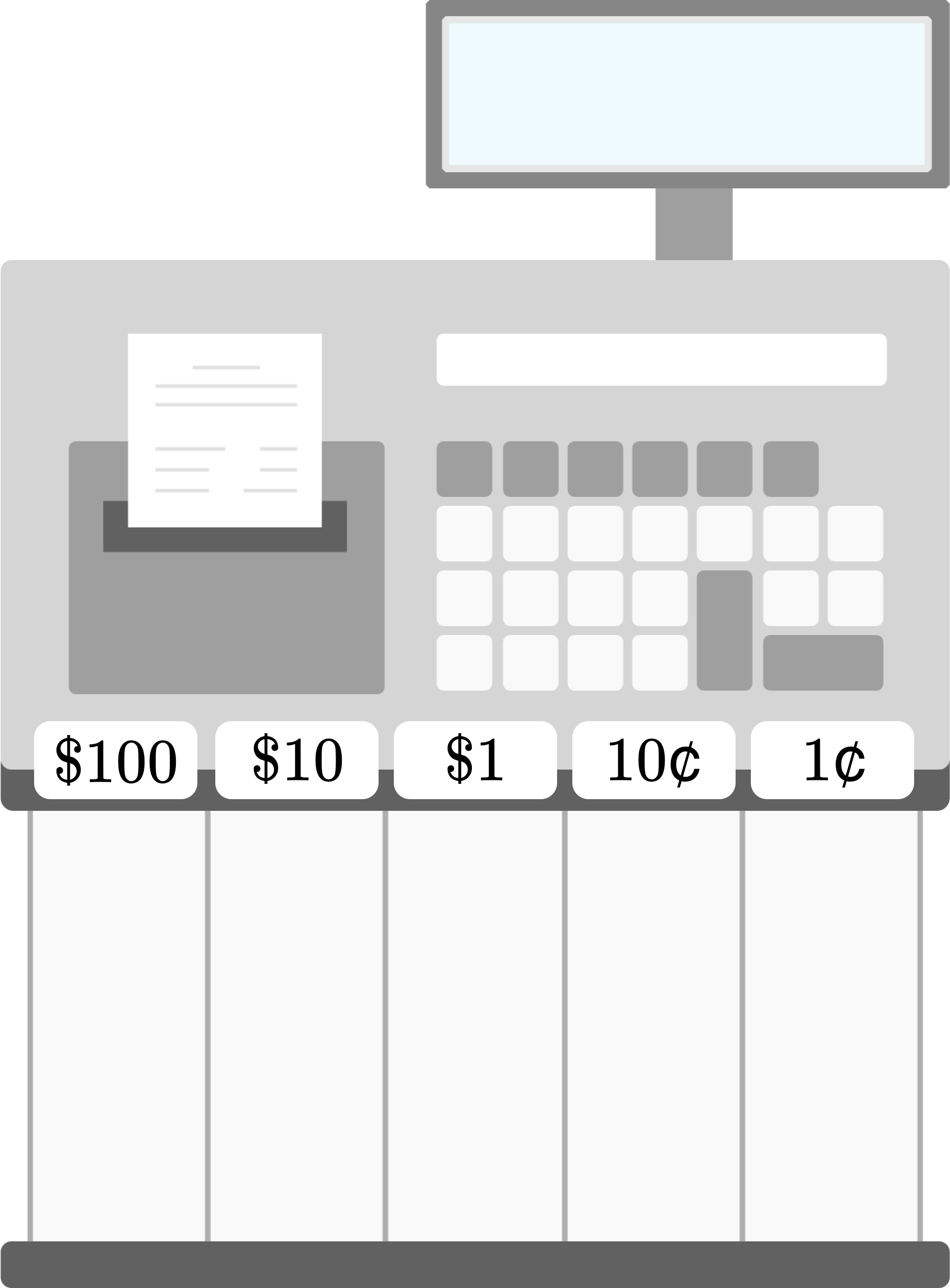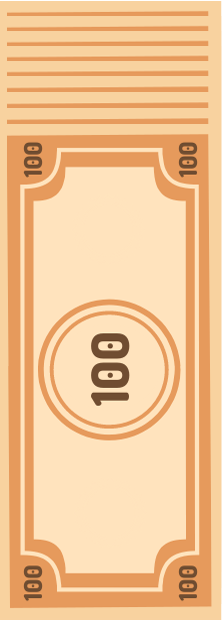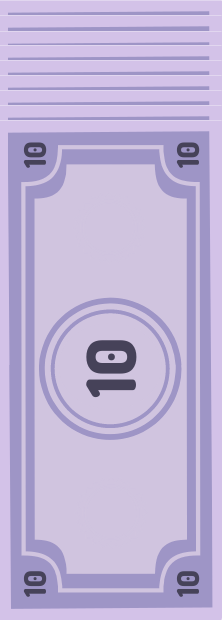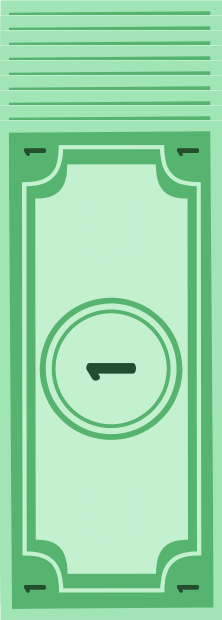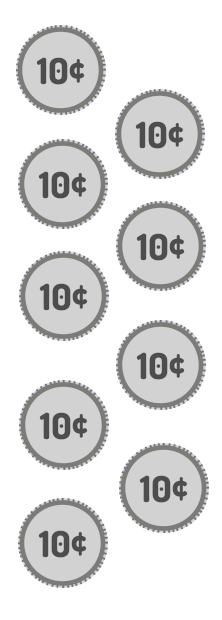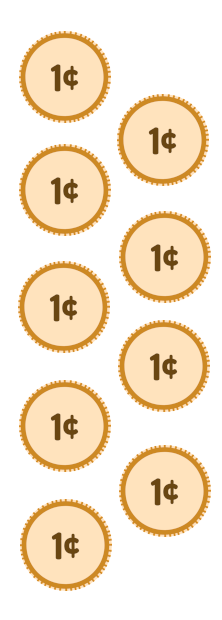Check out our
Calculator
or explore our
Lesson
and
Practice

CALCULATOR

KEY STEPS

## How to Read and Understand Decimals

### Step 1. Identify the value of the whole part.

This is like the number of dollars.

### Step 3. Identify the value of the decimal part.

This is like the number of cents.
LESSON

When we read decimals, we need to use our understanding of place values to identify the value of the whole part and the decimal part, which are separated by a decimal point.
millions
hundred-thousands
ten-thousands
thousands
hundreds
tens
ones
tenths
hundredths
thousandths
ten-thousandths
hundred-thousandths
millionths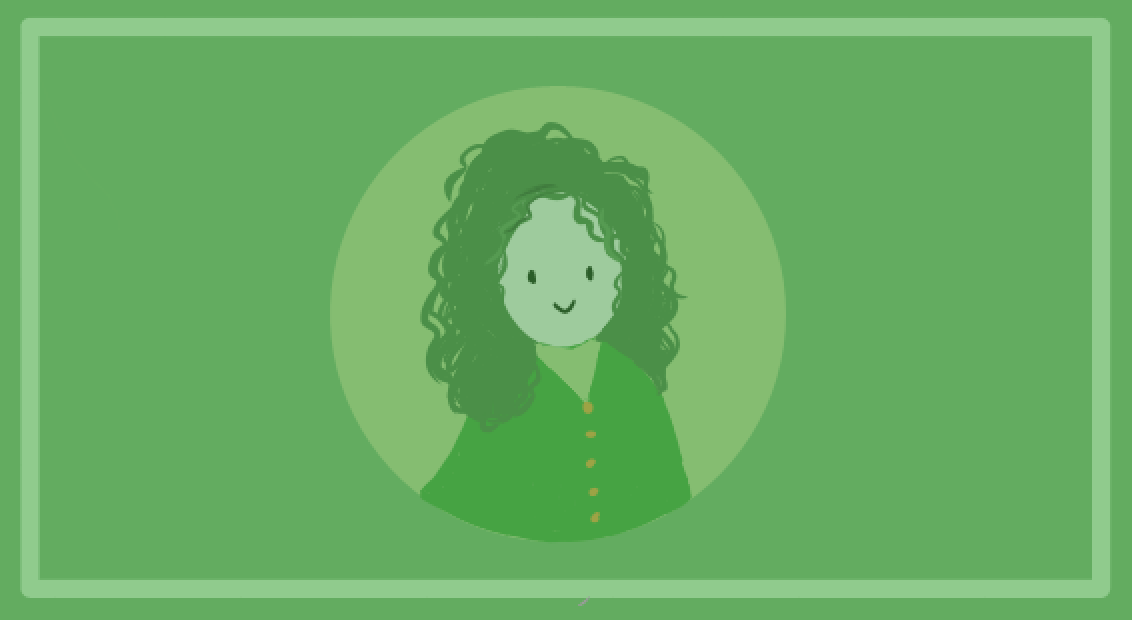decimal point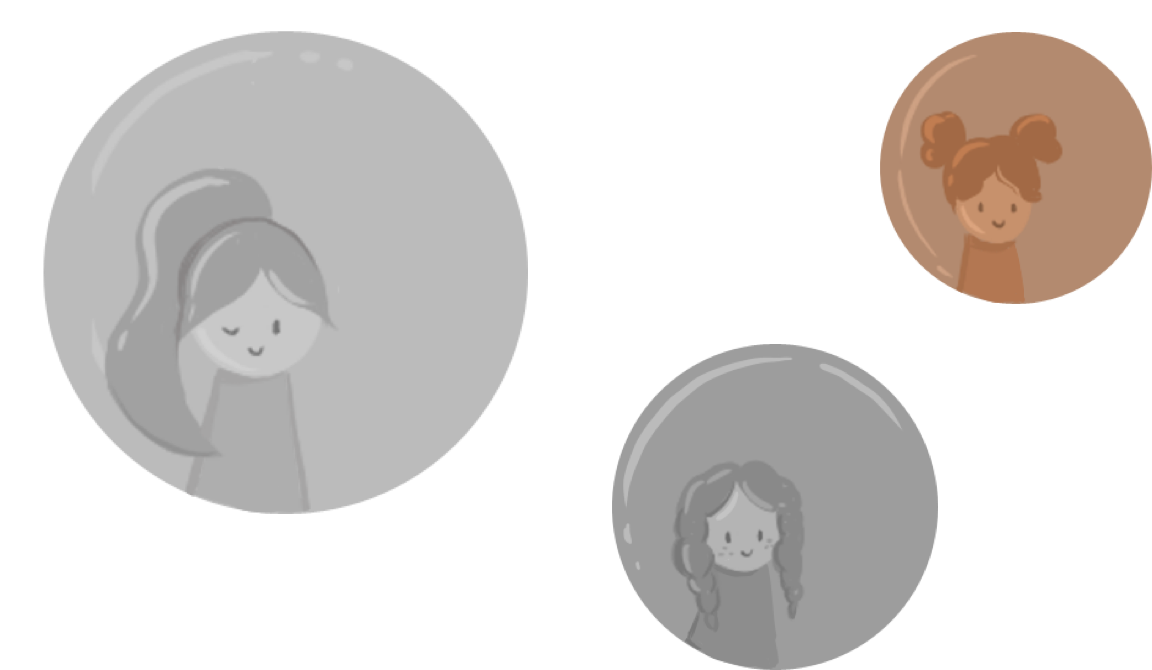Think of the decimal point as separating dollar bills from coins. Just like we would read as dollars and cents, we split up the whole part and the decimal part of a number:
 Whole Part Decimal Point Decimal Part Number Numerical Expansion Expansion hundred tens ones tenths hundredths thousandths In Words one hundred fifty-six and two hundred thirty-eight thousandths
Notice that in words, we read the decimal part as the number with the farthest decimal place value name, in this case ⬆️, thousandths.
Now that we know our basics, let’s check out some examples of how to understand decimals!
PRACTICE

## Practice: Reading and Understanding Decimals

Question 1 of 10: Interpret

### Step 1. Identify the value of the whole part.

This is like the number of dollars.decimal pointCONCLUSION
Incredible job, look at you go! Thanks for checking out this lesson ☺️🙏. Where to next?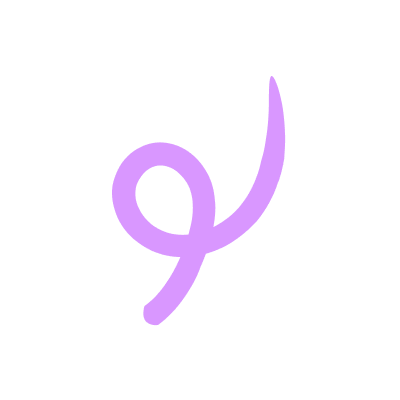Leave Feedback